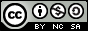- 目录 -
LeetCode 887 鸡蛋掉落(dp,记忆化搜索，二分)

输入：K = 1, N = 2



输入：K = 2, N = 6



输入：K = 3, N = 14



1. 1 <= K <= 100
2. 1 <= N <= 10000

## 思路

### 方法一：记忆化搜索+二分

dp[t][n]代表：有t层楼，n个鸡蛋，最少要扔多少次才可以确定临界值。现在定义一个k (1<=k<=t)，我们从第k层扔下去一个鸡蛋，如果鸡蛋碎了，我们就要往低一点的楼层去找，则当前dp[t][n]状态是dp[k-1][n-1]+1(k-1的原因是第k层已经扔过了)，如果鸡蛋没碎，我们要向上找，当前的状态dp[t][n]等价于dp[t-k][n]+1

• dp[k-1][n-1],k增大的时候，楼层越高，则值越大
• dp[t-k][n]，k增大的时候，楼层越小，值越小

## 方法二：dp

• dp[step]=0 : 0 个鸡蛋，不论走多少步，所能确定的最大楼层永远只是0
• dp[k]=1 ：不论有多少个鸡蛋，如果之走一步，那么只能确定最大1层楼。
• dp[step]=step：只有一个鸡蛋时，能走到的最大楼层只能1层一层试，需要step次数。

$$dp[k][step]=dp[k-1][step-1]+dp[k][step-1]+1$$

## 代码

  1 2 3 4 5 6 7 8 9 10 11 12 13 14 15 16 17 18 19 20 21 22 23 24 25 26 27 28 29  class Solution { public: // 返回有t层，n个鸡蛋的最小移动次数,k是枚举的中间楼层 int dp[10000 + 50][100 + 50]; int dfs(int t, int n) { if (~dp[t][n]) return dp[t][n]; if (t == 0 || n == 0) return 0; if (n == 1) return t; if (t == 1) return 1; int minn = INT_MAX; for (int k = 1; k <= t; k++) { minn = min(minn, max(dfs(k - 1, n - 1), dfs(t - k, n)) + 1); } return dp[t][n] = minn; } // K 代表鸡蛋个数，N 代表楼层数 int superEggDrop(int K, int N) { memset(dp, -1, sizeof(dp)); return dfs(N, K); } }; 

  1 2 3 4 5 6 7 8 9 10 11 12 13 14 15 16 17 18 19 20 21 22 23 24 25 26 27 28 29 30 31 32 33 34 35 36 37 38  class Solution { public: // 返回有t层，n个鸡蛋的最小移动次数,k是枚举的中间楼层 int dp[10000 + 50][100 + 50]; int dfs(int t, int n) { if (~dp[t][n]) return dp[t][n]; if (t == 0 || n == 0) return 0; if (n == 1) return t; if (t == 1) return 1; int l = 1, r = t; while (r - l > 1) { int mid = (l + r) >> 1; int ans1 = dfs(mid - 1, n - 1); int ans2 = dfs(t - mid, n); if (ans1 < ans2) l = mid; else if (ans1 > ans2) r = mid; else l = r = mid; } return dp[t][n] = 1 + min(max(dfs(l - 1, n - 1), dfs(t - l, n)), max(dfs(r - 1, n - 1), dfs(t - r, n))); } // K 代表鸡蛋个数，N 代表楼层数 int superEggDrop(int K, int N) { memset(dp, -1, sizeof(dp)); return dfs(N, K); } }; 

  1 2 3 4 5 6 7 8 9 10 11 12 13 14 15 16 17 18 19 20 21  class Solution { public: // K个鸡蛋，N层楼 int superEggDrop(int K, int N) { // dp[k][step]表示给k个鸡蛋，移动step步所能达到的最大层数 vector> dp(K + 1, vector(N + 1, 0)); for (int step = 1; step < N; step++) { dp[step] = 0; for (int k = 1; k <= K; k++) { dp[k][step] = dp[k][step - 1] + dp[k - 1][step - 1] + 1; if (dp[k][step] >= N) return step; } } return N; } };